# Correlation Coefficient using z-transformation

Hypothesis: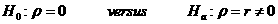Data Input: (Help) (Example)

 Input Results α β N r

Note:

 Variables Descriptions α Significance level (two sided test) 1-β Power of the test r Sample correlation N Sample size needed

Help Aids

Application:  To calculate the sample sizes needed to detect a relevant simple correlation with specified significance level and power, following hypotheses are usually used:Procedure:

1. Enter

a)    value of α, the probability of type I error

b)    value of β, the probability of type II error, or (1-power) of the test

c)    value of r, the sample correlation based on N observations.

1. Click the button “Calculate” to obtain the result sample size N needed for this hypothesis test.

Formula:

To employ Fisher’s arctanh transformation:Given a sample correlation r based on N observations that is distributed about an actual correlation value (parameter) ρ, then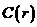is normally distributed with mean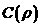and variance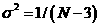.

Under the null hypothesis, the test statistic is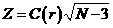where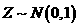The sample size to achieve specified significance level and power iswhere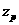is the upper 100(1-p) percentile of the standard normal distribution.

Notations:

α:         The probability of type I error (significance level) is the probability of rejecting the true null hypothesis.

β:         The probability of type II error (1 – power of the test) is the probability of not rejecting the false null hypothesis.

Example:

Suppose one wishes to detect a simple corrleation r (r=0.4) of N observations. Using a two sided test, 5% significance level test (α=0.05) with power 80% power (β=0.2), the required sample size is approximate 47 (n=47).

Reference: Lachin (1981) Controlled Clinical Trials 2: 93-113

Top